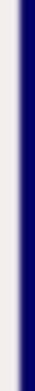homework help forum today's math problem links utilities previous math problem feedback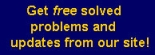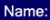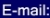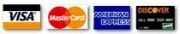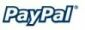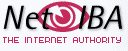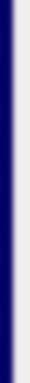# MATH HOMEWORK HELP

Problem: Given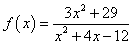(a) Find the equation of the vertical asymptotes, if any.

Solution: To find vertical asymptotes we look for divisions by 0. The denominator can be written as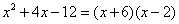The candidates to be asymptotes are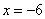and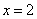. If we evaluate these values at the enumerator we get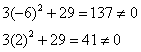This means that the vertical asymptotes areand.

(b) Find the equation of the horizontal asymptote, if any

Solution: We find thatTherefore,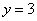is the unique horizontal asymptote.

MGT - Your source of Math and Statistics Homework Help